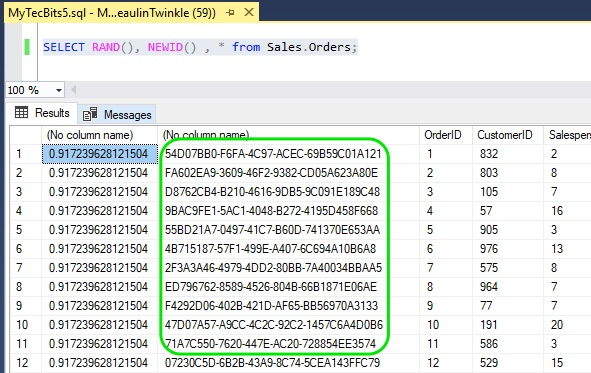# Generating Unique Random Number For Each Row in Select Statement

In my earlier article, I have discussed about generating random numbers in SQL Server using the RAND() math function. RAND is an easy way to generate random numbers. But, If you try to use RAND() in a select statement, you can see the same random number repeats in all the rows returned by the select query like this:

I am sure you are not expecting this, having the same random number on all the rows. So, how to overcome this problem and generate unique random number for every row in the result? There is a way to get separate random numbers for each row using NEWID. NEWID() is a uniqueidentifier with 16 byte hexadecimal digits. Here is an example:

Sometimes, this 16 byte unique identifier may not be useful for your situation. You may need random numbers in integer format. You may even need random numbers between two integers. In that case, you can CAST the NEWID() and apply a formula to get an random integer. Here is the syntax and example to generate random numbers between two integers using NEWID().

## Syntax

```ABS(CAST(CAST(NEWID() AS VARBINARY) AS INT)) % (@B - @A + 1) + @A

Where:
A = The smallest number of the range.
B = The largest number of the range.
```

## Example

This script will generate unique random number between two integer values (i.e between 70 and 100) for each row in the select statement result.

```Use WideWorldImporters;
DECLARE @A INT;
DECLARE @B INT;

SET @A = 70
SET @B = 100

SELECT Top 15
ABS(CAST(CAST(NEWID() AS VARBINARY) AS INT))
% (@B - @A + 1) + @A AS Random_Number,
* from Sales.Orders;
GO
```

## Reference

### Related Articles & Tools

This site uses Akismet to reduce spam. Learn how your comment data is processed.